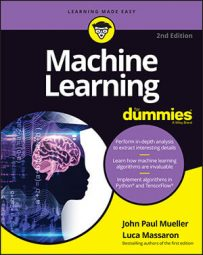##### Machine Learning For DummiesYou have a few possible strategies to handle missing data effectively for machine learning. Your strategy may change if you have to handle missing values in quantitative (values expressed as numbers) or qualitative features. Qualitative features, although also expressed by numbers, are in reality referring to concepts, so their values are somewhat arbitrary and you cannot meaningfully take an average or other computations on them.

When working with qualitative features, your value guessing should always produce integer numbers, based on the numbers used as codes. Common strategies for missing data handling are as follows:

• Replace missing values with a computed constant such as the mean or the median value. If your feature is a category, you must provide a specific value because the numbering is arbitrary, and using mean or median doesn’t make sense. Use this strategy when the missing values are random.
• Replace missing values with a value outside the normal value range of the feature. For instance, if the feature is positive, replace missing values with negative values. This approach works fine with decision tree–based algorithms and qualitative variables.
• Replace missing values with 0, which works well with regression models and standardized variables. This approach is also applicable to qualitative variables when they contain binary values.
• Interpolate the missing values when they are part of a series of values tied to time. This approach works only for quantitative values. For instance, if your feature is daily sales, you could use a moving average of the last seven days or pick the value at the same time the previous week.
• Impute their value using the information from other predictor features (but never use the response variable). Particularly in R, there are specialized libraries like missForest, MICE, and Amelia II that can do everything for you.

Another good practice is to create a new binary feature for each variable whose values you repaired. The binary variable will track variations due to replacement or imputing with a positive value, and your machine learning algorithm can figure out when it must make additional adjustments to the values you actually used.

In Python, missing values are made possible only using the ndarray data structure from the NumPy package. Python marks missing values with a special value that appears printed on the screen as NaN (Not a Number). The DataFrame data structure from the pandas package offers methods for both replacing missing values and dropping variables.

The following Python example demonstrates how to perform replacement tasks. It begins by creating a dataset of 5 observations and 3 features, named “A”, “B”, “C”:

`import pandas as pd`

`import numpy as np`

`data = pd.DataFrame([[1,2,np.nan],[np.nan,2,np.nan],`

`[3,np.nan,np.nan],[np.nan,3,8],`

`[5,3,np.nan]],columns=['A','B','C'])`

`print(data,'\n') # prints the data`

`# counts NaN values for each feature`

`print(data.isnull().sum(axis=0))`

` `

`A B C`

`0 1 2 NaN`

`1 NaN 2 NaN`

`2 3 NaN NaN`

`3 NaN 3 8`

`4 5 3 NaN`

` `

`A 2`

`B 1`

`C 4`

`dtype: int64`

Because feature C has just one value, you can drop it from the dataset. The code then replaces the missing values in feature B with a medium value and interpolates the value in feature A because it displays a progressive order.

`# Drops definitely C from the dataset`

`data.drop('C', axis=1, inplace=True)`

`# Creates a placeholder for B's missing values`

`data['missing_B'] = data['B'].isnull().astype(int)`

`# Fills missing items in B using B's average`

`data['B'].fillna(data['B'].mean(), inplace=True)`

`# Interpolates A`

`data['A'].interpolate(method='linear', inplace=True)`

`print(data)`

` `

`A B missing_B`

`0 1 2.0 0`

`1 2 2.0 0`

`2 3 2.5 1`

`3 4 3.0 0`

`4 5 3.0 0`

The printed output is the final dataset. Be sure to note that the mean of B isn’t an integer value, so the code converted all B values to floating numbers. This approach makes sense if B is numeric. If it were a category, and the numbering were marking a class, the code should have filled the feature using the command data['B'].fillna(data['B'].mode().iloc, inplace=True), which uses the mode, that is, the first most frequent value in the series.

In R, missing values appear marked as NA when printed or summarized. Both languages provide special ways to locate and deal with empty values. After you’ve located them, you have to decide whether to replace or remove them. To replicate the Python example in R, you need to install the zoo package for your platform to create interpolations:

`install.packages(pkgs='zoo', dependencies=TRUE)`

After installing the zoo package, you can create a data frame and replace the missing values using the same strategy as before:

`library(zoo)`

`df <- data.frame(A=c(1,NA,3,NA,5),`

`B=c(2,2,NA,3,3),`

`C=c(NA,NA,NA,8,NA))`

`print(df)`

` `

`A B C`

`1 1 2 NA`

`2 NA 2 NA`

`3 3 NA NA`

`4 NA 3 8`

`5 5 3 NA`

` `

`df <- subset(df, select = c('A','B'))`

`df['m_B'] <- as.numeric(is.na(df\$B))`

`df\$B[is.na(df\$B)] <- mean(df\$B, na.rm=TRUE)`

`df\$A <- na.approx(df\$A)`

`print(df)`

` `

`A B m_B`

`1 1 2.0 0`

`2 2 2.0 0`

`3 3 2.5 1`

`4 4 3.0 0`

`5 5 3.0 0`

As shown in the example, sometimes you can’t do much with examples that have a lot of missing values in their features. In such cases, if the example is for training (test examples should not be removed, instead), remove it from the set (a procedure called listwise deletion) so that the incomplete cases won’t affect learning. If instead, the example is part of your test, you shouldn’t remove it and use it to get an evaluation of how well your machine learning algorithm handles such situations.